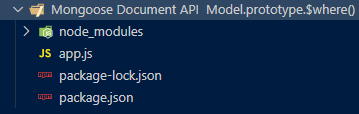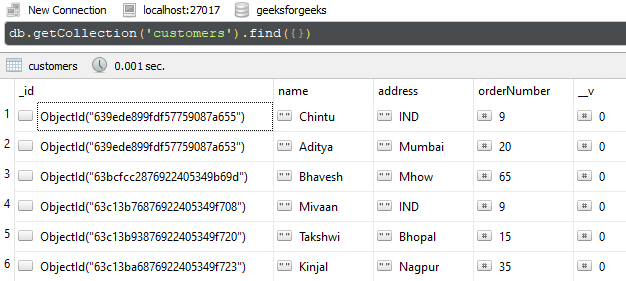Open in App
Not now

# Mongoose Document Model.prototype.\$where() API

• Last Updated : 24 Jan, 2023

The Mongoose Document API  Model.prototype.\$where() method of the Mongoose API is used on the Document model. It allows putting the where condition in the MongoDB query. Using this method we can form MongoDB query having JavaScript expression. We can use this method directly on the MongoDB model as well. Let us understand the \$where() method using an example.

Syntax:

`Model.\$where(<argument>);`

Parameters: This method accepts a single parameter as discussed below:

• argument: It is used to specify conditions using javascript expression or string or it can be a function.

Return Value: This method returns a query object on which we can call the callback function.

Setting up Node.js application:

Step 1: Create a Node.js application using the following command:

`npm init`

Step 2: After creating the NodeJS application, Install the required module using the following command:

`npm install mongoose`

Project Structure: The project structure will look like this:Database Structure: The database structure will look like this, the following documents are present in the collection.Example 1: In this example, we are illustrating the functionality of \$where() method. We are accessing the \$where() method directly on the Customer model and fetching the document where the name field’s value is Aditya.

Filename: app.js

## Javascript

 `// Require mongoose module ` `const mongoose = require(``"mongoose"``); ` ` `  `// Set Up the Database connection ` `const URI = ``"mongodb://localhost:27017/geeksforgeeks"``; ` ` `  `const connectionObject = mongoose.createConnection(URI, ` `{ ` `    ``useNewUrlParser: ``true``, ` `    ``useUnifiedTopology: ``true``, ` `}); ` ` `  `const Customer = connectionObject.model( ` `    ``"Customer"``, ` `    ``new` `mongoose.Schema({ ` `        ``name: String, ` `        ``address: String, ` `        ``orderNumber: Number, ` `    ``}) ` `); ` ` `  `Customer.\$where(``"this.name === 'Aditya'"``). ` `    ``then(res => { ` `    ``console.log(res); ` `}).``catch``(err => { ` `    ``console.log(err); ` `})`

Step to run the program: To run the application execute the below command from the root directory of the project:

`node app.js`

Output:

```[
{
_id: new ObjectId("639ede899fdf57759087a653"),
orderNumber: 20,
__v: 0
}
]```

Example 2: In this example, we are illustrating the functionality of \$where() method. We are accessing the \$where() form on the result set, and fetching the documents where the value of the orderNumber field is 9.

Filename: app.js

## Javascript

 `// Require mongoose module ` `const mongoose = require(``"mongoose"``); ` ` `  `// Set Up the Database connection ` `const URI = ``"mongodb://localhost:27017/geeksforgeeks"``; ` ` `  `const connectionObject = mongoose.createConnection(URI, { ` `    ``useNewUrlParser: ``true``, ` `    ``useUnifiedTopology: ``true``, ` `}); ` ` `  `const Customer = connectionObject.model( ` `    ``"Customer"``, ` `    ``new` `mongoose.Schema({ ` `        ``name: String, ` `        ``address: String, ` `        ``orderNumber: Number, ` `    ``}) ` `); ` ` `  `Customer.find().\$where((``"this.orderNumber === 9"``)). ` `    ``exec((error, result) => { ` `        ``if` `(error) { ` `            ``console.log(error); ` `        ``} ``else` `{ ` `            ``console.log(result); ` `        ``} ` `    ``})`

Step to run the program: To run the application execute the below command from the root directory of the project:

`node app.js`

Output:

```[
{
_id: new ObjectId("639ede899fdf57759087a655"),
name: 'Chintu',
orderNumber: 9,
__v: 0
},
{
_id: new ObjectId("63c13b76876922405349f708"),
name: 'Mivaan',